Study of mathematics online.
Study math with us and make sure that "Mathematics is easy!"

# Perimeter Formulas for Geometric Figures

Perimeter of a geometric figure
- is the continuous line forming the boundary of a closed geometrical figure.

## Perimeter of a triangle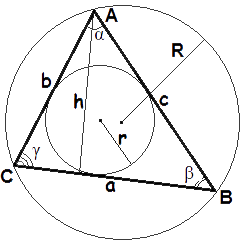The perimeter of the triangle ABC is the sum of the lengths of its sides

P = a + b + c

## Perimeter of a square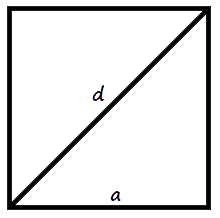The perimeter of a square is the product of the length of a square side by 4.

P = 4a

The perimeter of a square is the product of the length of a square diagonal by 2√2.

P = 2√2 d
where P - perimeter of a square,
a - the lenth of a square side,
d - the length of a square diagonal.

## Perimeter of a rectangle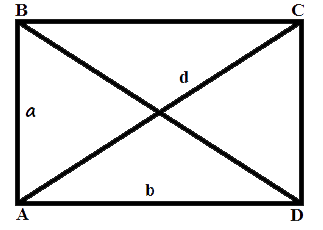The perimeter of the rectangle ABCD is equal to twice the sum of the sides adjacent to one corner.

P = 2(a + b)
where P - perimeter of a rectangle,
a, b - the length of rectangle sides,i.e., the height and width accordingly.

## Perimeter of a parallelogram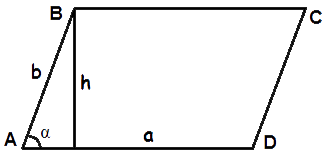Perimeter of the parallelogram ABCD is equal to twice the sum of the sides adjacent to one corner.

P = 2(a + b)
where P - perimeter of a parallelogram,
a, b - lengths of parallelogram sides.

## Perimeter of a rhombus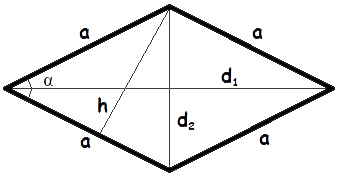The perimeter of a rhombus is equal to the product of the length of its side into four.

P = 4a
where P - perimeter of a rhombus,
a - the length of a rhombus side.

## Perimeter of a trapezoid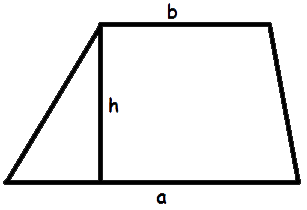The perimeter of a trapezoid is equal to the sum of the lengths of its sides.

P = a + b + c + d
where P - perimeter of a trapezoid,
a, b - the length of 2 trapezoid bases,i.e., the parallel sides,
c, d - length of the legs (the lateral sides).

## Perimeter of a circle (Circumference)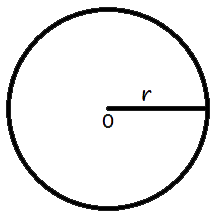P = 2 π r
P = π d
where P - perimeter of a circle,
r - the length of the circle radius,
d - the length of the circle diameter,
π = 3.141592.